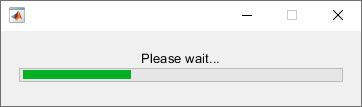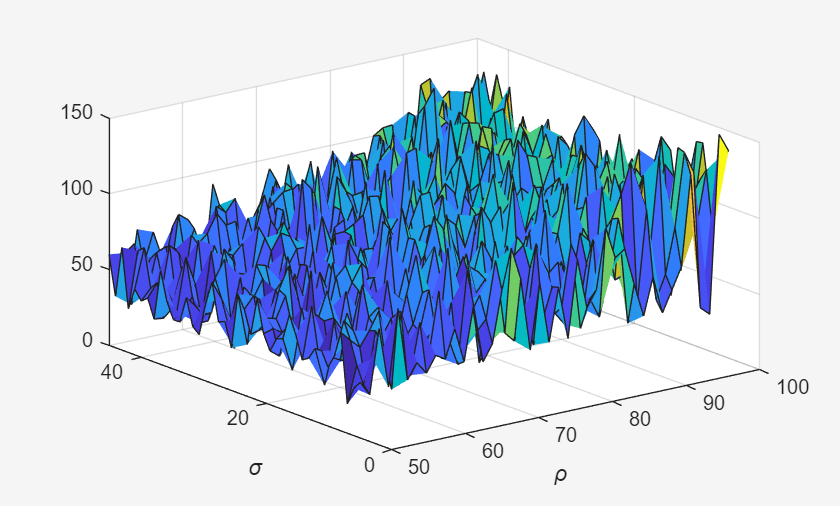# parallel.pool.DataQueue

Send and listen for data between client and workers

## Description

A DataQueue enables asynchronous sending data or messages from workers back to the client in a parallel pool while a computation is carried out. For example, you can get intermediate values and an indication of the progress of the computation.

To send data from a parallel pool worker back to the client, first construct a DataQueue in the client. Pass this DataQueue into a parfor-loop or other parallel language construct, such as spmd. From the workers, call send to send data back to the client. At the client, register a function to be called each time data is received by using afterEach.

• You can call send from the worker or client that creates the DataQueue, if required.

• You can construct the queue on the workers and send it back to the client to enable communication in the reverse direction. However, you cannot send a queue from one worker to another. To transfer data between workers, use spmd, spmdSend, or spmdReceive instead.

• Unlike all other handle objects, DataQueue and PollableDataQueue instances do remain connected when they are sent to workers.

## Creation

### Syntax

q = parallel.pool.DataQueue

### Description

example

q = parallel.pool.DataQueue creates an object that can be used to send or listen for messages (or data) from different workers. Create the DataQueue on the worker or client where you want to receive the data.

## Properties

expand all

This property is read-only.

The number of items of data waiting to be removed from the queue, specified as a zero or positive integer. The value is 0 or a positive integer on the worker or client that creates the PollableDataQueue instance. If the client creates the PollableDataQueue instance, the value is 0 on all workers. If a worker creates the PollableDataQueue, the value is 0 on the client and all other workers.

## Object Functions

 afterEach Define a function to call when new data is received on a DataQueue send Send data from worker to client using a data queue

## Examples

collapse all

Construct a DataQueue, and call afterEach.

q = parallel.pool.DataQueue; afterEach(q, @disp); 
Start a parfor-loop, and send a message. The pending message is passed to the afterEach function, in this example @disp.

parfor i = 1:3 send(q, i); end;
 1 2 3

For more details on listening for data using a DataQueue, see afterEach.

When you send a message to a DataQueue object, the message waits in the queue until it is processed by a listener. Each message adds 1 to the queue length. In this example, you use the QueueLength property to find the length of a DataQueue object.

When a client or worker creates a DataQueue object, any messages that are sent to the queue are held in the memory of that client or worker. If the client creates a DataQueue object, the QueueLength property on all workers is 0. In this example, you create a DataQueue object on the client, and send data from a worker.

First, create a parallel pool with one worker.

parpool(1);
Starting parallel pool (parpool) using the 'local' profile ... Connected to the parallel pool (number of workers: 1). 

Then, create a DataQueue.

q = parallel.pool.DataQueue
q = DataQueue with properties: QueueLength: 0 

A newly created DataQueue has an empty queue. You can use parfor to find q.QueueLength on the worker. Find the queue length on the client, and the queue length on the worker.

fprintf('On the client: %i\n', q.QueueLength)
On the client: 0 
parfor i = 1 fprintf('On the worker: %i\n', q.QueueLength) end
On the worker: 0 

As the queue is empty, the QueueLength is 0 for both the client and the worker. Next, send a message to the queue from the worker. Then, use the QueueLength property to find the length of the queue.

% Send a message first parfor i = 1 send(q, 'A message'); end % Find the length fprintf('On the client: %i\n', q.QueueLength)
On the client: 1 
parfor i = 1 fprintf('On the worker: %i\n', q.QueueLength) end
On the worker: 0 

The QueueLength property is 1 on the client, and 0 on the worker. Create a listener to process the queue by immediately displaying the data.

el = afterEach(q, @disp);

Wait until the queue is empty, then delete the listener.

while q.QueueLength > 0 pause(0.1); end delete(el);

Use the QueueLength property to find the length of the queue.

fprintf('On the client: %i\n', q.QueueLength)
On the client: 0 

QueueLength is 0 because the queue processing is complete.

In this example, you use a DataQueue to update a wait bar with the progress of a parfor-loop.

When you create a parfor-loop, you offload each iteration to workers in a parallel pool. Information is only returned from the workers when the parfor-loop completes. You can use a DataQueue to update a wait bar at the end of each iteration.

When you update a wait bar with the progress of your parfor-loop, the client must record information about how many iterations remain.

Tip

If you are creating new parallel code and want to monitor the progress of your code, consider using a parfeval workflow. For more information, see Update User Interface Asynchronously Using afterEach and afterAll.

The helper function parforWaitbar, defined at the end of this example, updates a wait bar. The function uses persistent to store information about the number of remaining iterations.

Use waitbar to create a wait bar, w.

w = waitbar(0,'Please wait ...');

Create a DataQueue, D. Then use afterEach to run parforWaitbar after messages are sent to the DataQueue.

% Create DataQueue and listener D = parallel.pool.DataQueue; afterEach(D,@parforWaitbar);

Set the number of iterations for your parfor-loop, N. Use the wait bar w and the number of iterations N to initialize the function parforWaitbar.

At the end of each iteration of the parfor-loop, the client runs parforWaitbar and incrementally updates the wait bar.

N = 100; parforWaitbar(w,N) 

The function parforWaitbar uses persistent variables to store the number of completed iterations on the client. No information is required from the workers.

Run a parfor-loop with N iterations. For this example, use pause and rand to simulate some work. After each iteration, use send to send a message to the DataQueue. When a message is sent to the DataQueue, the wait bar updates. Because no information is required from the workers, send an empty message to avoid unnecessary data transfer.

After the parfor-loop completes, use delete to close the wait bar.

parfor i = 1:N pause(rand) send(D,[]); end delete(w); 

Define the helper function parforWaitbar. When you run parforWaitbar with two input arguments, the function initializes three persistent variables (count, h, and N). When you run parforWaitbar with one input argument, the wait bar updates.

function parforWaitbar(waitbarHandle,iterations) persistent count h N if nargin == 2 % Initialize count = 0; h = waitbarHandle; N = iterations; else % Update the waitbar % Check whether the handle is a reference to a deleted object if isvalid(h) count = count + 1; waitbar(count / N,h); end end endThis example shows how to perform a parallel parameter sweep with parfeval and send results back during computations with a DataQueue object.

parfeval does not block MATLAB, so you can continue working while computations take place.

The example performs a parameter sweep on the Lorenz system of ordinary differential equations, on the parameters $\sigma$ and $\rho$, and shows the chaotic nature of this system.

$\begin{array}{l}\frac{\mathrm{d}}{\mathrm{d}\mathit{t}}\mathit{x}=\sigma \left(\mathit{y}-\mathit{z}\right)\\ \frac{\mathrm{d}}{\mathrm{d}\mathit{t}}\mathit{y}=\mathit{x}\left(\rho -\mathit{z}\right)-\mathit{y}\\ \frac{\mathrm{d}}{\mathrm{d}\mathit{t}}\mathit{z}=\mathit{xy}-\beta \mathit{x}\end{array}$

Create Parameter Grid

Define the range of parameters that you want to explore in the parameter sweep.

gridSize = 40; sigma = linspace(5, 45, gridSize); rho = linspace(50, 100, gridSize); beta = 8/3;

Create a 2-D grid of parameters by using the meshgrid function.

[rho,sigma] = meshgrid(rho,sigma);

Create a figure object, and set 'Visible' to true so that it opens in a new window, outside of the live script. To visualize the results of the parameter sweep, create a surface plot. Note that initializing the Z component of the surface with NaN creates an empty plot.

figure('Visible',true); surface = surf(rho,sigma,NaN(size(sigma))); xlabel('\rho','Interpreter','Tex') ylabel('\sigma','Interpreter','Tex')Set Up Parallel Environment

Create a pool of parallel workers by using the parpool function.

parpool;
Starting parallel pool (parpool) using the 'Processes' profile ... Connected to the parallel pool (number of workers: 6). 

To send data from the workers, create a DataQueue object. Set up a function that updates the surface plot each time a worker sends data by using the afterEach function. The updatePlot function is a supporting function defined at the end of the example.

Q = parallel.pool.DataQueue; afterEach(Q,@(data) updatePlot(surface,data));

Perform Parallel Parameter Sweep

After you define the parameters, you can perform the parallel parameter sweep.

parfeval works more efficiently when you distribute the workload. To distribute the workload, group the parameters to explore into partitions. For this example, split into uniform partitions of size step by using the colon operator (:). The resulting array partitions contains the boundaries of the partitions. Note that you must add the end point of the last partition.

step = 100; partitions = [1:step:numel(sigma), numel(sigma)+1]
partitions = 1×17 1 101 201 301 401 501 601 701 801 901 1001 1101 1201 1301 1401 1501 1601 

For best performance, try to split into partitions that are:

• Large enough that the computation time is large compared to the overhead of scheduling the partition.

• Small enough that there are enough partitions to keep all workers busy.

To represent function executions on parallel workers and hold their results, use future objects.

f(1:numel(partitions)-1) = parallel.FevalFuture;

Offload computations to parallel workers by using the parfeval function. parameterSweep is a helper function defined at the end of this script that solves the Lorenz system on a partition of the parameters to explore. It has one output argument, so you must specify 1 as the number of outputs in parfeval.

for ii = 1:numel(partitions)-1 f(ii) = parfeval(@parameterSweep,1,partitions(ii),partitions(ii+1),sigma,rho,beta,Q); end

parfeval does not block MATLAB, so you can continue working while computations take place. The workers compute in parallel and send intermediate results through the DataQueue as soon as they become available.

If you want to block MATLAB until parfeval completes, use the wait function on the future objects. Using the wait function is useful when subsequent code depends on the completion of parfeval.

wait(f);After parfeval finishes the computations, wait finishes and you can execute more code. For example, plot the contour of the resulting surface. Use the fetchOutputs function to retrieve the results stored in the future objects.

results = reshape(fetchOutputs(f),gridSize,[]); contourf(rho,sigma,results) xlabel('\rho','Interpreter','Tex') ylabel('\sigma','Interpreter','Tex')If your parameter sweep needs more computational resources and you have access to a cluster, you can scale up your parfeval computations. For more information, see Scale Up from Desktop to Cluster.

Define Helper Functions

Define a helper function that solves the Lorenz system on a partition of the parameters to explore. Send intermediate results to the MATLAB client by using the send function on the DataQueue object.

function results = parameterSweep(first,last,sigma,rho,beta,Q) results = zeros(last-first,1); for ii = first:last-1 lorenzSystem = @(t,a) [sigma(ii)*(a(2) - a(1)); a(1)*(rho(ii) - a(3)) - a(2); a(1)*a(2) - beta*a(3)]; [t,a] = ode45(lorenzSystem,[0 100],[1 1 1]); result = a(end,3); send(Q,[ii,result]); results(ii-first+1) = result; end end

Define another helper function that updates the surface plot when new data arrives.

function updatePlot(surface,data) surface.ZData(data(1)) = data(2); drawnow('limitrate'); end

## Version History

Introduced in R2017a